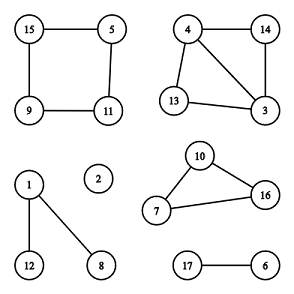# D - Cyclic Components

CodeForces - 977E

You are given an undirected graph consisting of nn vertices and mm edges. Your task is to find the number of connected components which are cycles.

Here are some definitions of graph theory.

An undirected graph consists of two sets: set of nodes (called vertices) and set of edges. Each edge connects a pair of vertices. All edges are bidirectional (i.e. if a vertex aa is connected with a vertex bb, a vertex bb is also connected with a vertex aa). An edge can’t connect vertex with itself, there is at most one edge between a pair of vertices.

Two vertices uu and vv belong to the same connected component if and only if there is at least one path along edges connecting uu and vv.

A connected component is a cycle if and only if its vertices can be reordered in such a way that:

• the first vertex is connected with the second vertex by an edge,
• the second vertex is connected with the third vertex by an edge,
• the last vertex is connected with the first vertex by an edge,
• all the described edges of a cycle are distinct.

A cycle doesn’t contain any other edges except described above. By definition any cycle contains three or more vertices.There are 66 connected components, 22 of them are cycles: [7,10,16][7,10,16] and [5,11,9,15][5,11,9,15].Input

The first line contains two integer numbers nn and mm (1≤n≤2⋅1051≤n≤2⋅105, 0≤m≤2⋅1050≤m≤2⋅105) — number of vertices and edges.

The following mm lines contains edges: edge ii is given as a pair of vertices vivi, uiui (1≤vi,ui≤n1≤vi,ui≤n, ui≠viui≠vi). There is no multiple edges in the given graph, i.e. for each pair (vi,uivi,ui) there no other pairs (vi,uivi,ui) and (ui,viui,vi) in the list of edges.Output

Print one integer — the number of connected components which are also cycles.ExamplesInput

5 4
1 2
3 4
5 4
3 5


Output

1


Input

17 15
1 8
1 12
5 11
11 9
9 15
15 5
4 13
3 13
4 3
10 16
7 10
16 7
14 3
14 4
17 6


Output

2


Note

In the first example only component [3,4,5][3,4,5] is also a cycle.

The illustration above corresponds to the second example.

#include<iostream>
#include<vector>
#include<queue>
#include<cstring>
#include<cmath>
#include<cstdio>
#include<algorithm>
using namespace std;
const int maxn=2e5+1000;
typedef long long LL;
LL n,m;
LL p[maxn];
vector<LL>g[maxn],c[maxn];
void init()
{
for(LL i=0;i<=n+3;i++) p[i]=i;
}
LL find(LL x)
{
if(x==p[x]) return p[x];
return p[x]=find(p[x]);
}
bool check(LL n)
{
for(LL i=0;i<c[n].size();i++)
{
if(g[c[n][i]].size()!=2)
return false;
}
return true;
}
int main(void)
{
cin.tie(0);std::ios::sync_with_stdio(false);
cin>>n>>m;
init();
while(m--)
{
LL x,y;cin>>x>>y;
g[x].push_back(y);
g[y].push_back(x);
p[find(x)]=find(y);
}

//找连通块
for(LL i=1;i<=n;i++)
{
c[find(i)].push_back(i);//划分连通块
}
LL ans=0;
for(LL i=1;i<=n;i++)
{
if(c[i].size()>2)//连通块里面的点数目>=3
{
if(check(i))
ans++;
}
}
cout<<ans<<endl;
return 0;
}
05-0896
04-176005-0734
05-14194
05-14286
12-1928
03-0827
08-0345
05-0799